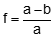### 2. Ellipsoid

An ellipse, Figure B-4, is a flattened circle having semi-major, a, and semi-minor, b, axes.Figure B-4 Ellipse

Geometric characteristics of an the ellipse are its flattening, f, first eccentricity, e, and second eccentricity, e'.Equation B-1Equation B-2Equation B-3

The ellipse is oriented so its semi-minor axis coincides with the Earth's polar axis, PP'. Spinning the ellipse around its semi-minor axis creates a 3D ellipsoid, Figure B-5.Figure B-5 Ellipsoid

An ellipsoid is used to build an earth model because it has the same general shape - bulging around the middle and flattened at top and bottom.